Create a new printableMath Worksheets

Sample - Click above to make a new math worksheet (PDF).
 Name _____________________________Date ___________________
Write the sum or difference.

1.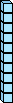-4 tens  -  2 tens

=  ___ tens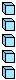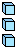-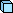8 ones  -  1 one

=  ___ ones

48  -  21  =  ______
2.-1 ten  -  1 ten

=  ___ tens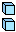-7 ones  -  6 ones

=  ___ ones

17  -  16  =  ______
3.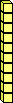+3 tens  +  1 ten

=  ___ tens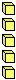+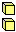5 ones  +  2 ones

=  ___ ones

35  +  12  =  ______
4.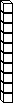-3 tens  -  2 tens

=  ___ tens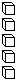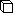-6 ones  -  6 ones

=  ___ ones

36  -  26  =  ______
 5 * This is a pre-made sheet.Use the link at the top of the page for a printable page.
6.-4 tens  -  1 ten

=  ___ tens-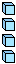5 ones  -  4 ones

=  ___ ones

45  -  14  =  ______